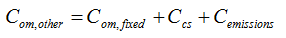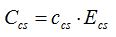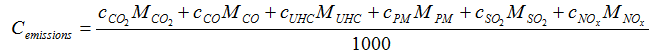# HOMER Pro 3.9

 Navigation: Glossary Other Operation and Maintenance CostType: Output Variable Units: \$/yr Symbol: Com,other

The other O&M cost is the sum of:

the system fixed O&M cost

the penalty for capacity shortage

the penalties for emissions of pollutants

HOMER uses the following equation to calculate the other O&M cost:where: Com,fixed = system fixed O&M cost [\$/yr] Ccs = the penalty for capacity shortage [\$/yr] Cemissions = the penalty for emissions [\$/yr]

HOMER uses the following equation to calculate the penalty for capacity shortage:where: ccs = capacity shortage penalty [\$/kWh] Ecs = total capacity shortage [kWh/yr]

HOMER uses the following equation to calculate the penalty for emissions:where: cCO2 = penalty for emissions of CO2 [\$/t] cCO = penalty for emissions of CO [\$/t] cUHC = penalty for emissions of unburned hydrocarbons (UHC) [\$/t] cPM = penalty for emissions of particulate matter (PM) [\$/t] cSO2 = penalty for emissions of SO2 [\$/t] cNOx = penalty for emissions of NOx [\$/t] MCO2 = annual emissions of CO2 [kg/yr] MCO = annual emissions of CO [kg/yr] MUHC = annual emissions of unburned hydrocarbons (UHC) [kg/yr] MPM = annual emissions of particulate matter (PM) [kg/yr] MSO2 = annual emissions of SO2 [kg/yr] MNOx = annual emissions of NOx [kg/yr]

You can specify the penalties for each pollutant on the Emissions window. For information on how HOMER calculates emissions of each pollutant, see the article on how HOMER calculates emissions.